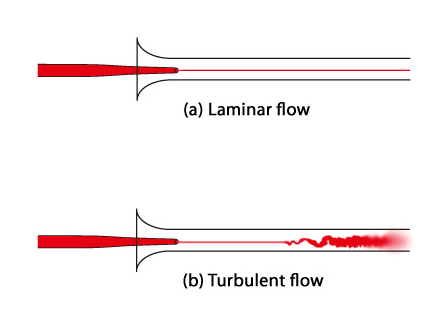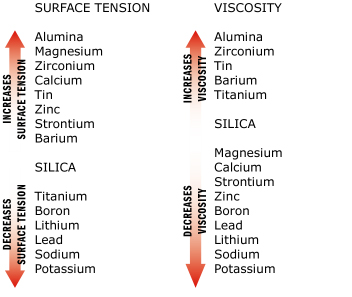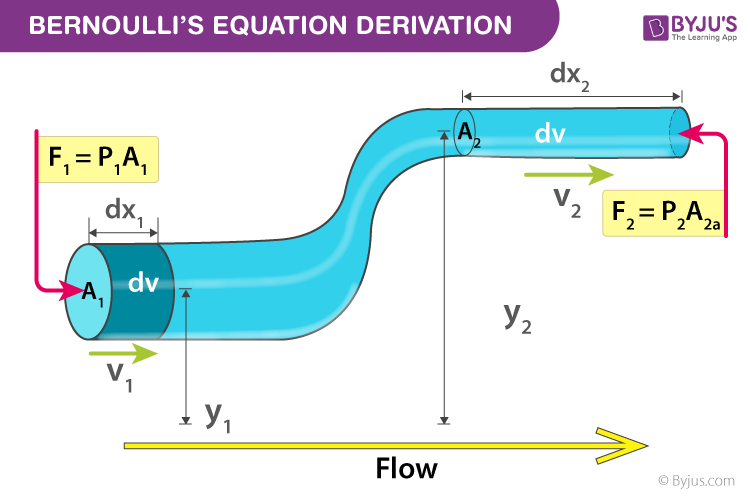# Mechanical Properties of Fluids

## What is Fluid?

Fluid is the name given to a substance which begins to flow when external force is applied on it.

Fluids possess characteristic physical properties that govern how they behave when forces are applied to them. Of particular interest are properties that govern fluid responses to ordinary mechanical forces; thus we will not consider electrical and magnetic phenomena.

## What are Mechanical Properties of Fluids?

The science of the mechanical properties of fluids is called Hydrostatics. A fluid is a substance which yields to the slightest pressure.

Fluids are divided into two classes distinguished by the names of liquids, and elastic fluids or gases, which later comprehends the air of the atmosphere and all the various kinds of air with which chemistry makes us acquainted. We shall confine our attention at present to the mechanical properties of liquids or non-elastic fluids.

Fluids show the effects of gravitation in a more perfect manner than solid bodies; the strong cohesive attraction of the particles of the latter in some measure counteracting the effect of gravity.

## Recommended Videos## Streamline Flow

A streamline is a curve the tangent to which at any point gives the direction of the fluid velocity at that point. It is analogous to a line of force in an electric or a magnetic field. In steady flow the pattern of streamline is stationary with time and therefore, a streamline gives the actual path of a fluid particle. A steady flow is therefore also called a streamlined flow or laminar flow. No two streamlines can ever cross one another, for if they did, a fluid particle arriving at that point could go one way or the other and the flow would not be steady.### Tube of Flow

A tubular region of fluid enclosed by a boundary consisting of streamlines is called a tube of flow. No fluid can cross the boundaries of a tube of flow and therefore, a tube of flow behaves like a pipe of the same shape.

## Surface Tension and Viscosity

The amount of force per unit length necessary to deform this interfacial surface is called the surface tension, and is denoted by the Greek letter sigma.

The surface tension of a liquid is always a function of the solid or fluid with which the liquid is in contact. If a value for surface tension is given in a table for oil, water, mercury, or whatever, and the contacting fluid is not specified, it is safe to assume that the contacting fluid is air.

The viscosity of a fluid is defined as the ratio of the shearing stress between adjacent layers of fluid to the rate of change of velocity perpendicular to the direction of motion. More simply stated viscosity if that property of a fluid which causes the fluid to resist flow. The higher the viscosity of a fluid the more it will resist flowing. The viscosity of most of the fluids we encounter in everyday life is independent of the applied external force.## Bernoulli’s Principle and Equation

Bernoulli’s Principle states that the total energy of the water always remains constant, therefore when the water flow in a system increases, the pressure must decrease. When water starts to flow in a hydraulic system the pressure drops. When the flow stops, the pressure rises again.In a hydraulic system, the total energy head is equal to the sum of three individual energy heads. This can be expressed as:

Where elevation head is the pressure due to the elevation of the water, pressure head is the height of a column of water that a given hydrostatic pressure in a system could support and velocity head is the energy present due to the velocity of the water.

## Pressure and Its Applications

All liquids and gases are fluids. The force exerted normally at a unit area of the surface of a fluid is called fluid pressure.

Consider a liquid which is confined in a closed vessel. Let the temperature of liquid is 20oC and pressure is atmospheric. This liquid will evaporate at 100oC. When vaporization takes place, the molecules escape from the free surface of the liquid. These vapour molecules get accumulated in the space between the free liquid surface and top of the vessel. These accumulated vapours exert a pressure on the liquid surface. Pressure is known as vapour pressure of the liquid. Or this is the pressure at which the liquid is converted into vapours.

## Frequently Asked Questions on Mechanical Properties of Fluids

### Is viscosity a mechanical property?

Viscosity is a fluid property, and a measure of flow resistance. In comparison, elasticity is a robust feature of the material. A viscoelastic material is therefore one which has both fluid and solid properties.

### What is streamline flow and turbulent flow?

Laminar and turbulent wind. Laminar flow or streamline flow in pipes (or tubes) occurs when a fluid flows in parallel layers, without any interruption between the layers. Turbulent flow is a flux system characterized by chaotic changes in properties. This involves quick variability in space and time of pressure and flow velocity.

### How does viscosity affect surface tension?

Surface tension is a contractive tendency of a liquid’s surface, which allows it to withstand external force. Viscosity increases with increase in temp but surface tension decreases. The finer the liquid would be the greater the viscosity. Both the viscosity and surface tension in a liquid diminish.

### Where is Bernoulli’s equation used?

Bernoulli’s equation is often used to include a speedometer called a pitot-static tube on aircrafts. For a mechanical tool a pressure is relatively easy to calculate. They evaluate the static and total pressure in a pitot-static tube and can then use Bernoulli’s equation to determine the velocity.

### What are the effects of fluid pressure?

Fluid pressure is determined by two factors. They are the fluid depth, and its density. At higher depths a fluid exerts greater pressure. Deeper into a fluid, any of the above fluid may allow more weight to push down.

Test your Knowledge on Mechanical properties of fluids!